Definitions of Square Dance Calls and Concepts

Index -->  Plus  |  A1  |  A2  |  C1  |  C2  |  C3A  |  C3B  |  C4  |  NOL  |
Definitions (Text Only) -->  Plus  |  A1  |  A2  |  C1  |  C2  |  C3A  |  C3B  |  C4  |  NOL  |
 Find call:

\$BJ?9T\$J(B Two-Faced Line \$B\$HB>\$NE,@Z\$J(B 2 x 4 formation (\$BNc\$(\$P8~\$+\$\$9g\$C\$?(B Line, \$BGXCf9g\$o\$;\$N(B Line, \$B\$=\$7\$F\$"\$k(B T-Bone) \$B\$+\$i!%(B

Ends \$B\$O(B Split Circulate \$B\$r(B, \$B30\$r8~\$\$\$?(B Centers \$B\$O(B Crossover Circulate \$B\$r(B, \$BCf\$r8~\$\$\$?(B Centers \$B\$O(B Concentric (Box) Crossover Circulate \$B\$r\$7\$^\$9!%(B

\$BJ?9T\$J(B Two-Faced Line \$B\$+\$i\$O(B, \$BJ?9T\$J(B Wave \$B\$G=*\$o\$j\$^\$9!%(B

Parallel Two-Faced Lines \$B\$+\$i(B: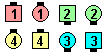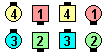Scatter Circulate\$B\$NA0(B \$B8e(B
T-Bone setup \$B\$+\$i(B: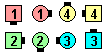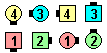Scatter Circulate\$B\$NA0(B \$B8e(B

\$BCm
• \$BCf\$r8~\$\$\$?(B centers \$B\$NF0\$-\$O(B, \$BIaDL(B Jaywalk \$B\$G9T\$o\$l\$F\$\$\$^\$9!%(B \$B\$7\$?\$,\$C\$F(B, \$BF0\$/A0\$K\$*8_\$\$\$K(B point \$B\$7\$J\$1\$l\$P\$J\$j\$^\$;\$s!%(B
• \$BJ?9T\$J(B Line \$B\$+\$i\$O(B, Scatter Circulate \$B\$O(B Stretched Box Circulate \$B\$HF1\$8\$G\$9!%(B
• Funny Scatter Circulate  (from Parallel 3 & 1 Lines)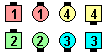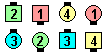Funny Scatter Circulate\$B\$NA0(B \$B8e(B

The above example is an instance of Funny where it is not those facing who do the call. The dancers who can move are those who can do the call in the normal manner without ending on the same spot as another dancer.

Go First Class [C4]: \$BJ?9T\$J(B Wave \$B\$^\$?\$OB>\$NE,@Z\$J(B formation \$B\$+\$i!%(B Leaders \$B\$,(B Switch the Wave \$B\$r\$9\$k4V\$K(B, Center Trailers \$B\$O(B Concentric (Box) Crossover Circulate \$B\$r(B, End Trailers \$B\$O(B Split Circulate \$B\$r\$7\$^\$9!%(B \$BJ?9T\$J(B Wave \$B\$+\$i\$O(B, \$BJ?9T\$J(B Two-Faced Line \$B\$G=*\$o\$j\$^\$9!%(B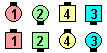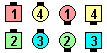Go First Class\$B\$NA0(B \$B8e(BChoreography for Scatter CirculateComments? Questions? Suggestions?